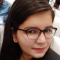## Recursive program to linearly search an element in a given array in C++Sunidhi Bansal

Updated on 03-Nov-2021 06:18:37

Given an integer array Arr[] containing integer numbers in any order. The goal is to find the input integer val present in the array using recursive search on the array.If val is not found in the input array Arr[] then return -1. Print the index of val if found in ... Read More

## Row-wise common elements in two diagonals of a square matrix in C++Sunidhi Bansal

Updated on 03-Nov-2021 06:15:22

Given a 2D square matrix as input. The goal is to find the elements that are common in both its primary and secondary diagonals. If the input matrix is1 2 3 2 2 4 1 4 7Then its primary diagonal is 1 2 7 and the secondary diagonal is 3 ... Read More

## Row-wise vs column-wise traversal of matrix in C++Sunidhi Bansal

Updated on 03-Nov-2021 06:08:30

A matrix can be traversed in two ways. Row-mise traversal visits each row one by one starting from first row then second and so on till the last row. Elements in the row are returned from index 0 to the last index.In Column-wise traversal, elements are traversed from the first ... Read More

## Reduce a number to 1 by performing given operations in C++Sunidhi Bansal

Updated on 03-Nov-2021 05:39:26

Given an integer Number as input. The goal is to find the minimum number of steps or operations required to reduce the input Number to 1. Operations that can be performed will be-:If Number is even, then Divide it by 2.If Number is odd, then increment or decrement it by ... Read More

## Reduce the fraction to its lowest form in C++Sunidhi Bansal

Updated on 03-Nov-2021 05:32:43

Given two integers Num1 and Num2 as input. The integers can be represented as fraction Num1/Num2. The goal is to reduce this fraction to its lowest form.Using GCD to find highest denominatorWe will calculate the greatest common divisor of both numbers.Divide both numbers by that gcdSet both variables as quotient ... Read More

## Reduce the array to a single integer with the given operation in C++Sunidhi Bansal

Updated on 03-Nov-2021 05:30:11

Given an integer variable Number as input. Let us consider an array containing elements in the range 1 to Number in any order. If we perform an operation Number-1 times on an array such thatWe select two elements A and B from the arrayRemove A and B from arrayAdd sum ... Read More

## Reduce the array to a single element with the given operation in C++Sunidhi Bansal

Updated on 03-Nov-2021 05:26:04

Given an integer variable Number as input. Let us consider an array containing elements in the range 1 to Number in sorted order. If we perform an operation on an array such that at each step the elements at odd positions are removed. Then the goal is to perform this ... Read More

## C Program to Redeclaration of global variableSunidhi Bansal

Updated on 03-Nov-2021 05:22:57

We will understand how C and C++ behave differently in case we re-declare a global variable without initializing, redeclaring global variables with initialization, redeclaring global variables and initializing them twice. Also, we will repeat above combinations with local variables.1. A) C program : Redeclaring Global variables with no initialization#include ... Read More

## Recursive Selection Sort in C++Sunidhi Bansal

Updated on 03-Nov-2021 05:14:49

Selection Sort is one of the sorting algorithms used to sort data by iterating an array from the beginning and replacing each element with the smallest element in the list. As we move forward the left array is sorted, and the right array is unsorted. Each move places the next ... Read More

## Reverse a number using stack in C++Sunidhi Bansal

Updated on 03-Nov-2021 05:11:32

We are given an integer number Num as input. The goal is to find the reverse of the number using stack.Stack:- A stack is a data structure in C++ which stores data in LIFO ( Last in First Out) manner. Major operations of stack are-:Declaration-: stack stck; //stck is ... Read More